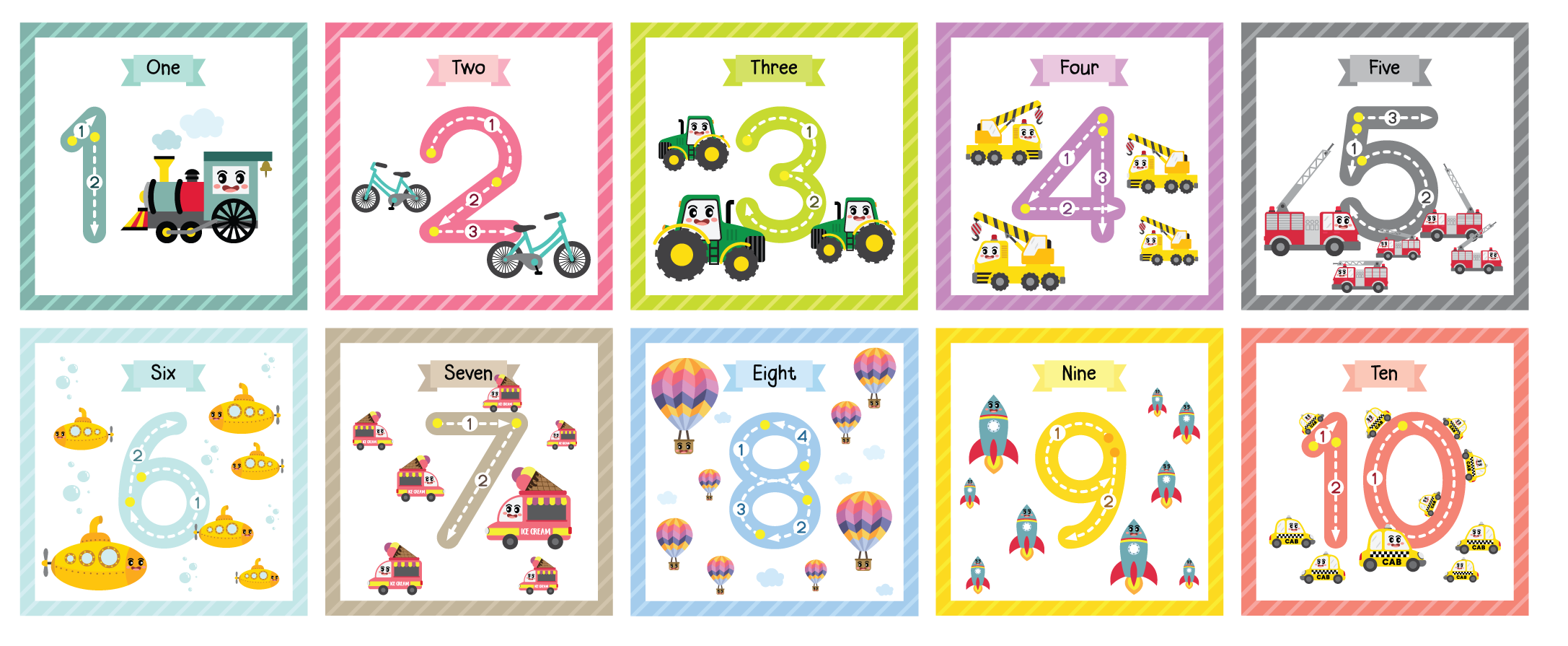Counting from 1 to 10 is really fun!

Try and remember the spelling for each number:

• 1 = One
• 2 = Two
• 3 = Three
• 4 = Four
• 5 = Five
• 6 = Six
• 7 = Seven
• 8 = Eight
• 9 = Nine
• 10 = TenAre you ready? 1..2..3.. let’s start the activities!